April 22, 2021, 12:12:26 pm
 Welcome, Guest. Please login or register. 1 Hour 1 Day Forever Login with username, password and session length
 News: Welcome to Wolf Scaper 317 siteHome Help Arcade Gallery HIGHSCORES Staff List Login Register

# The Basics.

 Pages:    Go DownAuthor Topic: The Basics.  (Read 367 times)
Wolf Scaper
Administrator
wolf-scaper legend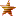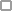Offline

Posts: 100046« on: March 29, 2010, 01:45:59 pm »

Well, I figured many people would possibly like to join the Wolf Scaper Development team some day.  So, I noticed when I talk about java, not many people have any idea what I'm talking about, thus, I am creating this topic just to teach people that basics of java.

The Basics, Lesson 1:

First things first: variables. Java has several fundamental variable types, known as 'primitives'.
These variable types include:

* boolean
* char
* byte
* short
* int
* long
* float
* double

Each of these primitives can store data. More information on primitive data types can be found here:

http://java.sun.com/docs/books/tutorial/java/nutsandbolts/datatypes.html

I suggest you read the information found on this page before continuing with this tutorial. If you are too lazy to do that then you are probably best off leaving the forums - we don't want you here.

Now that you know how to store data, you're probably wanting to work with it. This is when operators become useful; they allow you to do all sorts of things with variables: you can compare variable, you can increment them, decrement them, and so on. I will omit unary operators, for the sake of keeping this tutorial simple for newcomers.
The basic operators are as follows:

* Equality operators: != and ==
* Increment operator: ++
* Decrement operator: --
* Multiplication operator: *
* Division operator: /
* Addition operator: +
* Subtraction operator: -
* Logical AND operator: &&
* Logical OR operator: ||
* Relational LESS THAN: <
* Relational GREATER THAN: >
* Relational LESS THAN OR EQUAL TO: <=
* Relational GREATER THAN OR EQUAL TO: >=
* Assignment operators: +=, -=, *=, /=

The aforementioned operators allow you to change the value of a variable. Let's take a look at how these work.

Presume x has a value of 5, lets say this is the timer for a minigame.  To begin with in each of the examples below:
Examples   New x value
x++;   x equals 6(Adding Seconds)
x--;   x equals 4(Counting Down Seconds)
x += 5;   x equals 10(Adding 5 Seconds)
x -= 5;   x equals 0(Subtracting 5 Seconds)
x *= 5;   x equals 25(Multiplying Seconds By 5)
x /= 5;   x equals 1(Dividing Seconds By 5)

Now, a simple way to look at this is, we would only use the adding seconds and counting down seconds method on a minigame or anything that tracks time.

Now that we have learned to assign new values to a variable, we can learn how to compare variables to other variables.
Let's look at some boolean expressions based on comparing two ints, x and y.

For this example x will have a value of 5, and y will have a value of 10:
Examples   Returned value
x < y;   Returns TRUE(This is an operator saying that 5 is less than 10, this is true so the statement is true.)

x > y;   Returns FALSE(This is an operator saying 5 is greater than 10, this is false, so the statement is false.)

y <= x;   Returns FALSE(This is an operator saying that 10 is less than or equal to 5, this is not true so the statement is false.)

x >= y;   Returns FALSE(This is an operator saying that 5 is greater than or equal to 10, this is not true so the statement is false.)

x == y;   Returns FALSE(This is for INT, == just means equals, so when it says 5 == 10, it means 5 = 10, and that is true, so the statement is false)

x != y;   Returns TRUE(When it says != this mean Does NOT Equal, as we can see...5 does not equal 10, therefore the statement is false)

x == 5;   Returns TRUE(This is for an INT, == just means equals, so when it says 5 == 5, it means 5 = 5, and that is true, so the statement is true)

x != 5;   Returns FALSE(When it says != this mean Does NOT Equal, as we can see...5 does equal 5, therefore the statement is false)

It is important for you to understand this table so that you can pick up on understanding the basics of coding commands, minigames, timers, process's, and also sockets.

Now, lets move into a little harder section.

Lets make a basic command.

client plr = p.server.playerHandler.getPlayerID[ID];
if(command.startsWith("update")){
if(plr.ID && absX >= 10000 && absX <= 10000 && absY >= 10000 && absY <= 10000){
plr.setWalkableInterface(1999);
systemUpdateTime--;
}

This is basically saying, when you type ::update, the yellow text in the corner shows up for everyone that is within the reach, the reach here is set to, X 0, Y 0, X 10000, Y 10000.  This basically mean, wherever they are, they will get the update screen.

Then, the setWalkableInterface, this just means that when they walk the interface will not close.

Then the, systemUpdateTime--; if you apply the knowledge you learned above, this means that the timer is counting down.

Now we shall make the update packet.

if(systemUpdateTimer == 0){
plr.commandEqualsIgnoreCase("Kick All");
./compile/
./run/
}

This means that when the update timer hits 0, everyone will get kicked and the server will compile and restart, thus installing the update.

Well, thats about all I have to teach you about basic Java.  If you wish to join the development team, post back like this:

Code:
Name:
In Game Name:
Age:
Files You Want To Code:
Coding Experience, any/none:
How Long You Are On:
 Report SpamLoggedCheck out my thread on Free Runescape Bots for more information.

pieplom
Jr. Member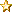Offline

Gender: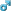Posts: 57Hello, you like pancakes??« Reply #1 on: June 26, 2010, 01:43:41 am »

Well, I figured many people would possibly like to join the Wolf Scaper Development team some day.  So, I noticed when I talk about java, not many people have any idea what I'm talking about, thus, I am creating this topic just to teach people that basics of java.

The Basics, Lesson 1:

First things first: variables. Java has several fundamental variable types, known as 'primitives'.
These variable types include:

* boolean
* char
* byte
* short
* int
* long
* float
* double

Each of these primitives can store data. More information on primitive data types can be found here:

http://java.sun.com/docs/books/tutorial/java/nutsandbolts/datatypes.html

I suggest you read the information found on this page before continuing with this tutorial. If you are too lazy to do that then you are probably best off leaving the forums - we don't want you here.

Now that you know how to store data, you're probably wanting to work with it. This is when operators become useful; they allow you to do all sorts of things with variables: you can compare variable, you can increment them, decrement them, and so on. I will omit unary operators, for the sake of keeping this tutorial simple for newcomers.
The basic operators are as follows:

* Equality operators: != and ==
* Increment operator: ++
* Decrement operator: --
* Multiplication operator: *
* Division operator: /
* Addition operator: +
* Subtraction operator: -
* Logical AND operator: &&
* Logical OR operator: ||
* Relational LESS THAN: <
* Relational GREATER THAN: >
* Relational LESS THAN OR EQUAL TO: <=
* Relational GREATER THAN OR EQUAL TO: >=
* Assignment operators: +=, -=, *=, /=

The aforementioned operators allow you to change the value of a variable. Let's take a look at how these work.

Presume x has a value of 5, lets say this is the timer for a minigame.  To begin with in each of the examples below:
Examples   New x value
x++;   x equals 6(Adding Seconds)
x--;   x equals 4(Counting Down Seconds)
x += 5;   x equals 10(Adding 5 Seconds)
x -= 5;   x equals 0(Subtracting 5 Seconds)
x *= 5;   x equals 25(Multiplying Seconds By 5)
x /= 5;   x equals 1(Dividing Seconds By 5)

Now, a simple way to look at this is, we would only use the adding seconds and counting down seconds method on a minigame or anything that tracks time.

Now that we have learned to assign new values to a variable, we can learn how to compare variables to other variables.
Let's look at some boolean expressions based on comparing two ints, x and y.

For this example x will have a value of 5, and y will have a value of 10:
Examples   Returned value
x < y;   Returns TRUE(This is an operator saying that 5 is less than 10, this is true so the statement is true.)

x > y;   Returns FALSE(This is an operator saying 5 is greater than 10, this is false, so the statement is false.)

y <= x;   Returns FALSE(This is an operator saying that 10 is less than or equal to 5, this is not true so the statement is false.)

x >= y;   Returns FALSE(This is an operator saying that 5 is greater than or equal to 10, this is not true so the statement is false.)

x == y;   Returns FALSE(This is for INT, == just means equals, so when it says 5 == 10, it means 5 = 10, and that is true, so the statement is false)

x != y;   Returns TRUE(When it says != this mean Does NOT Equal, as we can see...5 does not equal 10, therefore the statement is false)

x == 5;   Returns TRUE(This is for an INT, == just means equals, so when it says 5 == 5, it means 5 = 5, and that is true, so the statement is true)

x != 5;   Returns FALSE(When it says != this mean Does NOT Equal, as we can see...5 does equal 5, therefore the statement is false)

It is important for you to understand this table so that you can pick up on understanding the basics of coding commands, minigames, timers, process's, and also sockets.

Now, lets move into a little harder section.

Lets make a basic command.

client plr = p.server.playerHandler.getPlayerID[ID];
if(command.startsWith("update")){
if(plr.ID && absX >= 10000 && absX <= 10000 && absY >= 10000 && absY <= 10000){
plr.setWalkableInterface(1999);
systemUpdateTime--;
}

This is basically saying, when you type ::update, the yellow text in the corner shows up for everyone that is within the reach, the reach here is set to, X 0, Y 0, X 10000, Y 10000.  This basically mean, wherever they are, they will get the update screen.

Then, the setWalkableInterface, this just means that when they walk the interface will not close.

Then the, systemUpdateTime--; if you apply the knowledge you learned above, this means that the timer is counting down.

Now we shall make the update packet.

if(systemUpdateTimer == 0){
plr.commandEqualsIgnoreCase("Kick All");
./compile/
./run/
}

This means that when the update timer hits 0, everyone will get kicked and the server will compile and restart, thus installing the update.

Well, thats about all I have to teach you about basic Java.  If you wish to join the development team, post back like this:

Code:
Name:
In Game Name:
Age:
Files You Want To Code:
Coding Experience, any/none:
How Long You Are On:
Ok, i've read it, but i still don't get it.
 Report SpamLogged

Sausages and smoking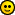myo biz
new memberOffline

Posts: 5« Reply #2 on: June 27, 2010, 06:59:06 pm »

u dont get it because your thinking to hard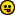nah jk umm when u start messing around with making servers its not that hard to understand.
 Report SpamLogged
Damuel
Jr. MemberOffline

Gender:Posts: 77« Reply #3 on: July 14, 2010, 03:02:51 pm »

Ok, i've read it, but i still don't get it.

Java isn't for everyone.
 Report SpamLoggedPages:    Go Up
Jump to:

Page created in 0.078 seconds with 18 queries.